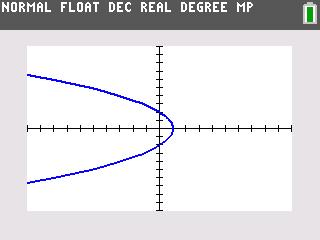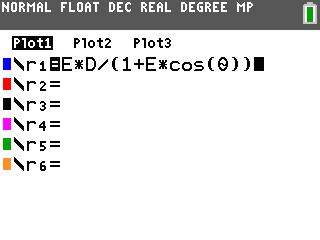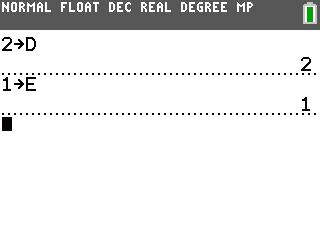# Activities

••• ##### Subject Area

• Math: Precalculus: Analytic Geometry (Conics)

• ##### Author9-12

60 Minutes

• ##### Device
• TI-83 Plus Family
• TI-84 Plus
• TI-84 Plus Silver Edition
•TI-84 Plus C Silver Edition
•TI-84 Plus CE
• ##### Report an Issue

Conics In Winter#### Activity Overview

Students explore conic graphing using a polar notation equation and determine the effects the various variables on the graph.

#### Key Steps

•Students explore how the eccentricity affects the graph of a conic section in polar coordinates. They enter the general polar equation for a conic section in Y=.

•Before viewing the graph, students initialize the variables. They set D = 2 and E = 1.

•They will see that for a parabola the eccentricity is 1, for a hyperbola the absolute value of the eccentricity is greater than 1, and the absolute value of the eccentricity of an ellipse is less than 1.